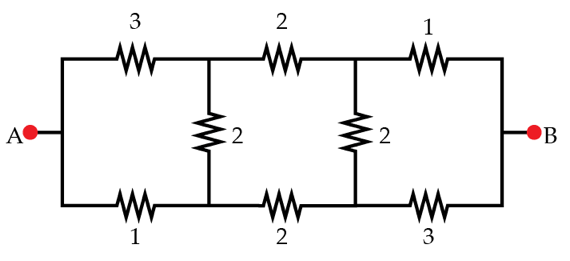# A problem on equivalent resistance

Determine the resistance in ohms between the points A and B (equivalent resistance) of the circuit shown below. All the values of the resistances are given in ohms.×